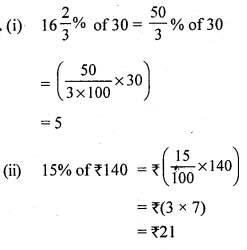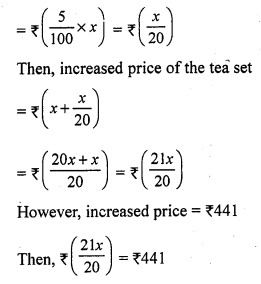# RS Aggarwal Class 7 Solutions Chapter 10 Percentage CCE Test Paper

In this chapter, we provide RS Aggarwal Solutions for Class 7 Chapter 10 Percentage CCE Test Paper for English medium students, Which will very helpful for every student in their exams. Students can download the latest RS Aggarwal Solutions for Class 7 Chapter 10 Percentage CCE Test Paper Maths pdf, free RS Aggarwal Solutions Class 7 Chapter 10 Percentage CCE Test Paper Maths book pdf download. Now you will get step by step solution to each question.

### RS Aggarwal Solutions for Class 7 Chapter 10 Percentage CCE Test Paper Download PDF

Question 1.
Solution:
We have :Question 2.
Solution:
(i) Let x% of 1 kg be 125 gQuestion 3.
Solution:Question 4.
Solution:
(i) Let x be the required number.Question 5.
Solution:
Let x be the number
The number is increased by 10%
Increased number = 110% of xQuestion 6.
Solution:
The present value of the machine = ₹ 10000
The decrease in its value after the 1 st year = 10% of ₹ 10000
10100 x 10000 = ₹ 1000
The depreciated value of the machine after the 1 st year = ₹ (10000 – 1000) = ₹ 9000
The decrease in its value after th 2nd year = 10% of ₹ 9000
10100 x 9000 = 900
The depreciated value of the machine after the 2nd year = ₹ (9000 – 900) = ₹ 8100
Hence, the value of the machine after two years will be ₹ 8100.

Question 7.
Solution:
The present population of the town = 16000
Increase in population after 1 year = 5% of 16000
= (5100 x 16000) = 800
Thus, population after one year = 16000 + 800 = 16800
Increase in population after 2 years = 5% of 16800
= 5100 x 16800 = 840
Population after two years = 16800 + 840 = 17640
Hence, the population of the town after two years will be 17,640.

Question 8.
Solution:
Let us assume that the original price of the tea set is Increase in price = 5%
So, value increased on the tea set = 5% of ₹ xHence, the original price of the tea set is ₹ 420.

Mark (✓) against the correct answer in each of the following :
Question 9.
Solution:Question 10.
Solution:
(c) 12
Given that x% of 75 = 12Question 11.
Solution:
(c) 25
Let the number be x. Then, we have:
120% of x = increased numberQuestion 12.
Solution:
(d) 180
Let the required number be x.
Then, we have :
5% of x = 9Question 13.
Solution:
(a) 60
Let the number be x According to question, we get:
(35 % of x) + 39 = xQuestion 14.
Solution:
(c) 500
Let x be the maximum marks Pass marks = (160 + 20) = 180
36 % of x = 180Question 15.
Solution:
(i) 3 : 4 = (75) %
Explanation:
3 : 4 = 34
= (34 x 100) %
= (3 x 25)% = 75%
(ii) 0.75 = (75)%
Explanation : (0.75 x 100)% = 75%
(iii) 6% = 0.06 (express in decimals)
Explanation :
6% = 6100 = 0.06
(iv) If x decreased by 40% gives 135, then x = 225
Explanation :
Let the number be x.
According to question, we have :
x – 40% of x = 135Question 16.
Solution:
(i) True (T)All Chapter RS Aggarwal Solutions For Class 7 Maths

—————————————————————————–

All Subject NCERT Exemplar Problems Solutions For Class 7

All Subject NCERT Solutions For Class 7

*************************************************

I think you got complete solutions for this chapter. If You have any queries regarding this chapter, please comment on the below section our subject teacher will answer you. We tried our best to give complete solutions so you got good marks in your exam.

If these solutions have helped you, you can also share rsaggarwalsolutions.in to your friends.